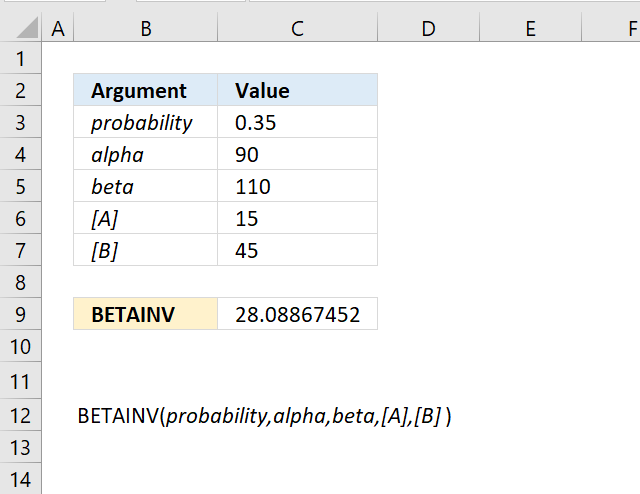Author: Oscar Cronquist Article last updated on September 24, 2018The BETAINV function calculates the inverse of the beta distribution. This function is outdated and replaced by the BETA.INV function which was introduced in Excel 2010.

Formula in cell C10:

=BETAINV(C3,C4,C5,C6,C7)

### Excel Function Syntax

BETAINV(probability,alpha,beta,[A],[B])

### Arguments

 probability Required. alpha Required. A parameter which determines the shape of the distribution. beta Required. A parameter which determines the shape of the distribution. [A] Optional. Lower bound, default value 0 (zero). [B] Optional. Upper bound, default value 1.